français | español | polski | 日本語 |

Pokemon xtra math teacher 1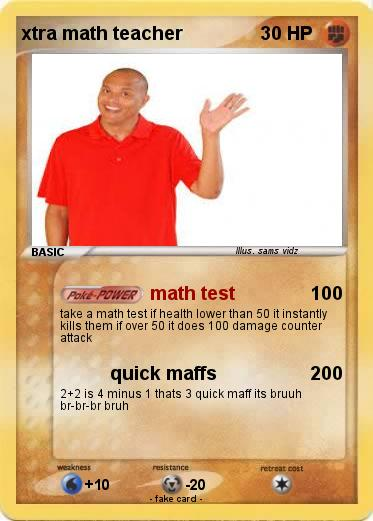Language Card : english

Creation Date : 8 January 2020

### Pokemon Passport

Name : xtra math teacher

Serie : 1st generation

Type : Fighting

Attack 1 : math test
take a math test if health lower than 50 it instantly kills them if over 50 it does 100 damage counter attack

Attack 2 : quick maffs
2+2 is 4 minus 1 thats 3 quick maff its bruuh br-br-br bruh

Illustrator : sams vidz

### Vote for this card

(1 vote)

Related cards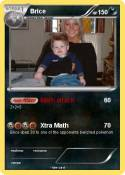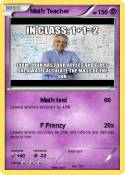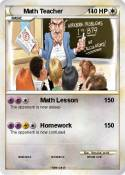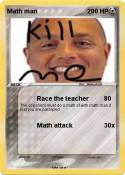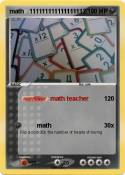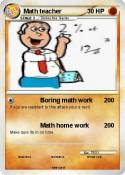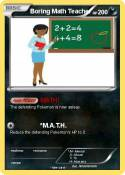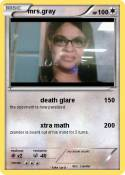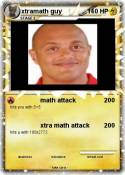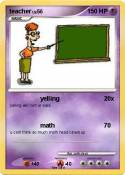Teacher | teacher | Mrs.Gerl | Teacher | boring teacher | Vote if you like pi! | teacher | Teacher | math teacher | math teacher | Xtramath Guy | Xtramath Guy 3 | teacher | your math teacher | Evil Math Teacher | The teacher | Best Teacher | Hue | Math teacher | Math Teacher | Gregs math teacher | Evil math teacher | Teacher Magic | Evil Teacher's brain | xtra op guy | Awsome-teacher | Vote 5 if you like math | Vote if u H8 MATH bad as diz face | Math Lover | Im too young for math | Mrs.Spinks | Angry Teacher | Dabbing Teacher | math mega | Sinapore Math 3B | JiJi ST math | The Great Math Wolf | Foxeh the math Fox | Khan academy | Vote 5 if you hate math | Math Mann | Ratey the Math Cat | I Can Do Math Everyday! | MATH | MATh | math team | Math Book | Math knight | Math | Math :( | math 100 x | me in math | Daniel EX | Albert Einstein | MATH | MATH | MS.WILLOUBY | Nerd | math | Math Homework | Cool Math | math doesn't lie | Tushar | Math 24 | Math Knight | Math Man | Math | Math | Mrs Ferriola | 1+1